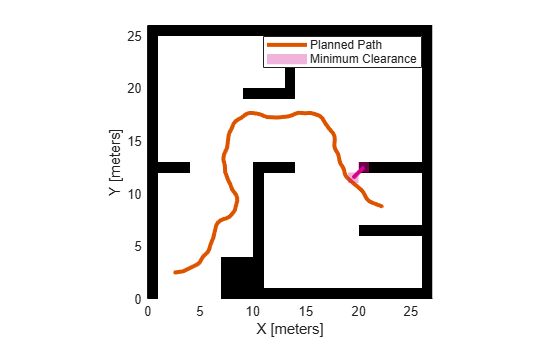# show

Visualize path metrics in map environment

## Syntax

``show(pathMetricsObj)``
``show(pathMetricsObj,Name,Value)``
``axHandle = show(pathMetricsObj)``

## Description

example

````show(pathMetricsObj)` plots the path in the map environment with the minimum clearance.```
````show(pathMetricsObj,Name,Value)` specifies additional options using one or more name-value pair arguments.```
````axHandle = show(pathMetricsObj)` outputs the axes handle of the figure used to plot the path.```

## Examples

collapse all

Compute smoothness, clearance, and validity of a planned path based on a set of poses and the associated map environment.

Load and Assign Map to State Validator

Create an occupancy map from an example map and set the map resolution.

```load exampleMaps.mat; % simpleMap mapResolution = 1; % cells/meter map = occupancyMap(simpleMap,mapResolution);```

Create a Dubins state space.

`statespace = stateSpaceDubins;`

Create a state validator based on occupancy map to store the parameters and states in the Dubins state space.

`statevalidator = validatorOccupancyMap(statespace);`

Assign the map to the validator.

`statevalidator.Map = map;`

Set the validation distance for the validator.

`statevalidator.ValidationDistance = 0.01;`

Update the state space bounds to be the same as the map limits.

`statespace.StateBounds = [map.XWorldLimits;map.YWorldLimits;[-pi pi]];`

Plan Path

Create an RRT* path planner and allow further optimization.

```planner = plannerRRTStar(statespace,statevalidator); planner.ContinueAfterGoalReached = true;```

Reduce the maximum number of iterations and increase the maximum connection distance.

```planner.MaxIterations = 2500; planner.MaxConnectionDistance = 0.3;```

Define start and goal states for the path planner as `[`x, y, theta`]` vectors. x and y are the Cartesian coordinates, and theta is the orientation angle.

```start = [2.5, 2.5, 0]; % [meters, meters, radians] goal = [22.5, 8.75, 0];```

Plan a path from the start state to the goal state. The plan function returns a `navPath` object.

```rng(100,'twister') % repeatable result [path,solutionInfo] = plan(planner,start,goal);```

Compute and Visualize Path Metrics

Create a path metrics object.

`pathMetricsObj = pathmetrics(path,statevalidator);`

Check path validity. The result is `1 (true)` if the planned path is obstacle free. `0 (false)` indicates an invalid path.

`isPathValid(pathMetricsObj)`
```ans = logical 1 ```

Calculate the minimum clearance of the path.

`clearance(pathMetricsObj)`
```ans = 0.21 ```

Evaluate the smoothness of the path. Values close to `0` indicate a smoother path. Straight-line paths return a value of `0`.

`smoothness(pathMetricsObj)`
```ans = 2.21 ```

Visualize the minimum clearance of the path.

```show(pathMetricsObj) legend('Planned Path','Minimum Clearance')```## Input Arguments

collapse all

Information for path metrics, specified as a `pathmetrics` object.

### Name-Value Pair Arguments

Specify optional comma-separated pairs of `Name,Value` arguments. `Name` is the argument name and `Value` is the corresponding value. `Name` must appear inside quotes. You can specify several name and value pair arguments in any order as `Name1,Value1,...,NameN,ValueN`.

Example: `'Parent',axHandle`

Axes used to plot path, specified as the comma-separated pair consisting of `'Parent'` and either an `axes` or `uiaxes` object. If you do not specify `Parent`, a new figure is created.

Example: `show(pathMetricsObj,'Parent',axHandle)`

Display metrics option, specified as the comma-separated pair consisting of `'Metrics'` and a cell array with any combination of these values:

• `'MinClearance'` — Display minimum clearance of path.

• `'StatesClearance'` — Display clearance of path states.

• `'Smoothness'` — Display path smoothness.

Example: `show(pathMetricsObj,'Metrics',{'Smoothness','StatesClearance'})`

Data Types: `cell`

## Output Arguments

collapse all

Axes used to plot path, returned as either an `axes` or `uiaxes` object.

### Functions

#### Introduced in R2019b